# Principal Stresses and Directions Civil Engineering (CE) Notes | EduRev

## Civil Engineering (CE) : Principal Stresses and Directions Civil Engineering (CE) Notes | EduRev

The document Principal Stresses and Directions Civil Engineering (CE) Notes | EduRev is a part of the Civil Engineering (CE) Course Advanced Solid Mechanics - Notes, Videos, MCQs & PPTs.
All you need of Civil Engineering (CE) at this link: Civil Engineering (CE)

Principal stresses and directions
At a given location in the body the magnitude of the normal and shear traction depend on the orientation of the plane. An isotropic body can fail along any plane and hence we require to find the maximum magnitude of these normal and shear traction and the planes for which these occur. As we shall see on planes where the maximum or minimum normal stresses occur the shear stresses are zero. However, in the plane on which maximum shear stress occurs, the normal stresses will exist.

Maximum and minimum normal traction
In order to obtain the maximum and minimum values of σn, the normal traction, we have to find the orientation of the plane in which this occurs. This is done by maximizing (4.5) subject to the constraint that | n | = 1. This constrained optimization is done by what is called as the Lagrange-multiplier method. Towards this, we introduce the function

L(n, λ*) = n · σn − λ* [| n |2 −1],                         (4.10)

where λ* is the Lagrange multiplier and the condition | n |2 − 1 = 0 characterizes the constraint condition. At locations where the extremal values of L occurs, the derivatives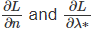must vanish, i.e.,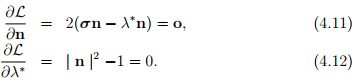To obtain these equations we have made use of the fact that Cauchy stress tensor is symmetric. Thus, we have to find three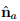and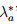’s such that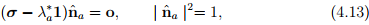(a = 1, 2, 3; no summation) which is nothing but the eigenvalue problem involving the tensor σ with the Lagrange multiplier being identified as the eigenvalue. Hence, the results of section 2.5 follows. In particular, for (4.13a) to have a non-trivial solution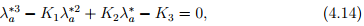where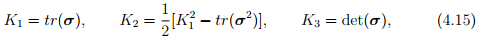the principal invariants of the stress σ. As stated in section 2.5, equation (4.14) has three real roots, since the Cauchy stress tensor is symmetric. These roots () will henceforth be denoted by σ1, σ2 and σ3 and are called principal stresses. The principal stresses include both the maximum and minimum normal stresses among all planes passing through a given x.
The corresponding three orthonormal eigenvectors, which are then characterized through the relation (4.13) are called the principal directions of σ. The planes for which these eigenvectors are normal are called principal planes. Further, these eigenvectors form a mutually orthogonal basis since the stress tensor σ is symmetric. This property of the stress tensor also allows us to represent σ in the spectral formIt is immediately apparent that the shear stresses in the principal planes are zero. Thus, principal planes can also be defined as those planes in which the shear stresses vanish. Consequently, σa’s are normal stresses.

Maximum and minimum shear traction
Next, we are interested in finding the direction of the unit vector n at x that gives the maximum and minimum values of the shear traction, τp. This is important because in many metals the failure is due to sliding of planes resulting due to the shear traction exceeding a critical value along some plane. In the following we choose the eigenvectors {} of σ as the set of basis vectors. Then, according to the spectral decomposition (4.16), all the nondiagonal matrix components of the Cauchy stress vanish. Then, the traction vector t(p) on an arbitrary plane with normal p could simply be written as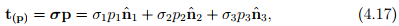where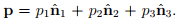It then follows from (4.9) that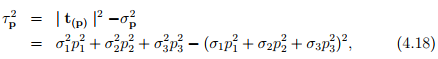where σp is the normal traction on the plane whose normal is p.
With the constraint condition | p |2 = 1 we can eliminate p3 from the equation (4.18). Then, since the principal stresses, σ1, σ2, σ3 are known,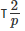is a function of only p1 and p2. Therefore to obtain the extremal values ofwe differentiatewith respect to p1 and p2 and equate it to zero1 :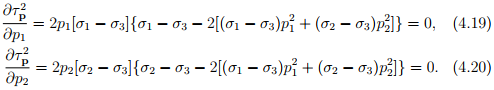The above set of equations has three classes of solutions.

• Set - 1: p1 = p2 = 0 and hence p3 = ±1, obtained from the condition that | p |2 = 1.

• Set - 2: p1 = 0, p2 = ±1/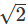and hence p= ±1/• Set - 3: p1 = ±1/, p2 = 0 and hence p3 = ±1/Instead of eliminating p3 we could eliminate p2 or pinitially and find the remaining unknowns by adopting a procedure similar to the above. Then, we shall find the extremal values of τp could also occur when

• Set - 4: p1 = p3 = 0 and hence p2 = ±1.

• Set - 5: p2 = p3 = 0 and hence p1 = ±1.

• Set - 6: p3 = 0, p1 = ±1/and hence p2 = ±1/.

1Recognize that the extremal values of both τp andoccur at the same location.

Substituting these solutions in (4.18) we find the extremal values of τp. Thus, τp = 0 when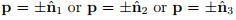and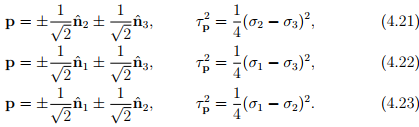Consequently, the maximum magnitude of the shear traction denoted by τmax is given by the largest of the three values of (4.21b) - (4.23b). Thus, we obtain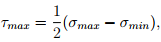(4.24)

where σmax and σmin denote the maximum and minimum magnitudes of principal stresses, respectively. Recognize that the maximum shear stress acts on a plane that is shifted about an angle of ±45 degrees to the principal plane in which the maximum and minimum principal stresses act. In addition, we can show that the normal traction σp to the plane in which τmax occurs has the value σp = (σmax + σmin)/2.

Offer running on EduRev: Apply code STAYHOME200 to get INR 200 off on our premium plan EduRev Infinity!

## Advanced Solid Mechanics - Notes, Videos, MCQs & PPTs

42 videos|61 docs

,

,

,

,

,

,

,

,

,

,

,

,

,

,

,

,

,

,

,

,

,

;